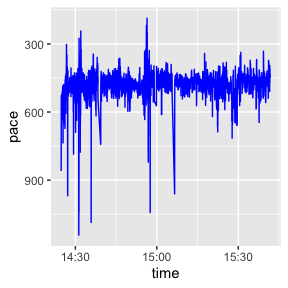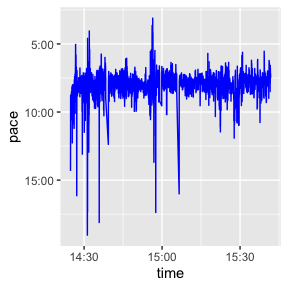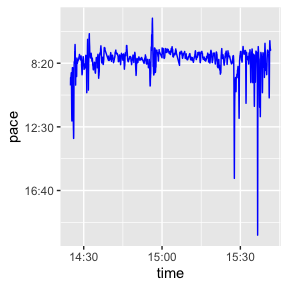# Handling Speed and Pace with activatr

#### 2021-01-11

activatr has utility functions to easily annotate a GPX DF with information about the speed (and corresponding pace) of an activity.

First, let’s parse our sample GPX DF:

library(activatr)

# Get the running_example.gpx file included with this package.
filename <- system.file(
"extdata",
"running_example.gpx.gz",
package = "activatr")

df <- parse_gpx(filename)
lat lon ele time
37.80405 -122.4267 17.0 2018-11-03 14:24:45
37.80406 -122.4267 16.8 2018-11-03 14:24:46
37.80408 -122.4266 17.0 2018-11-03 14:24:48
37.80409 -122.4266 17.0 2018-11-03 14:24:49
37.80409 -122.4265 17.2 2018-11-03 14:24:50

This has information about location and time, but it’s often useful to visualize the “mile pace” over the course of an activity, especially for runs.

The first thing to add to the table is the speed. mutate_with_speed is a wrapper around dyplr::mutate that will add a speed column to the data frame:

df <- mutate_with_speed(df)
lat lon ele time speed
37.80405 -122.4267 17.0 2018-11-03 14:24:45 NA
37.80406 -122.4267 16.8 2018-11-03 14:24:46 1.872248
37.80408 -122.4266 17.0 2018-11-03 14:24:48 2.253735
37.80409 -122.4266 17.0 2018-11-03 14:24:49 2.897343
37.80409 -122.4265 17.2 2018-11-03 14:24:50 3.052919

However, speed (in meters per second) isn’t the most useful metric for runs; mile pace (in minutes and seconds) is a more common measurement. We can convert speed to pace using speed_to_mile_pace:

df$pace <- speed_to_mile_pace(df$speed)
lat lon ele time speed pace
37.80405 -122.4267 17.0 2018-11-03 14:24:45 NA NA
37.80406 -122.4267 16.8 2018-11-03 14:24:46 1.872248 859.576124978957s (~14.33 minutes)
37.80408 -122.4266 17.0 2018-11-03 14:24:48 2.253735 714.076761340002s (~11.9 minutes)
37.80409 -122.4266 17.0 2018-11-03 14:24:49 2.897343 555.45378682477s (~9.26 minutes)
37.80409 -122.4265 17.2 2018-11-03 14:24:50 3.052919 527.147986777459s (~8.79 minutes)

Finally, while pace here is a lubridate duration object, it’s most easily understood in a “MM:SS” format: pace_formatter is provided for that: contrast the readability of the two pace graphs below, one without the formatter and the other with it:

library(ggplot2)
library(dplyr)
library(lubridate)
ggplot(filter(df, as.numeric(pace) < 1200)) +
geom_line(aes(x = time, y = as.numeric(pace)), color = "blue") +
scale_y_reverse() +
ylab("pace")
ggplot(filter(df, as.numeric(pace) < 1200)) +
geom_line(aes(x = time, y = as.numeric(pace)), color = "blue") +
scale_y_reverse(label=pace_formatter) +
ylab("pace")Note that the speed and pace are calculated at every point, so they will often be somewhat noisy. Computing the rolling mean of the speed to smooth out the graph would be numerically inaccurate, so mutate_with_speed provides helper arguments to allow the speed to be computed over larger windows.

In particular, it has lag and lead (which default to 1 and 0 respectively), which determines the “start” and “end” points used for the speed computation. So if we wanted each point’s speed to be determined using the points ten ahead and ten behind and plot that, we could do:

df <- mutate_with_speed(df, lead = 10, lag = 10)
df$pace <- speed_to_mile_pace(df$speed)
ggplot(filter(df, as.numeric(pace) < 1200)) +
geom_line(aes(x = time, y = as.numeric(pace)), color = "blue") +
scale_y_reverse(label=pace_formatter) +
ylab("pace")